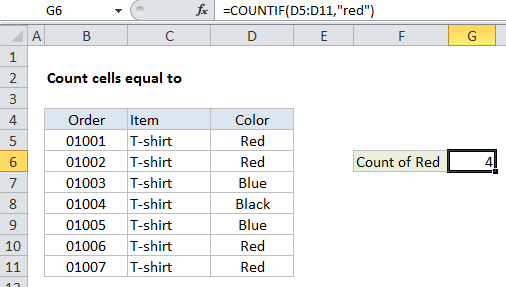## Excel Office

Excel How Tos, Tutorials, Tips & Tricks, Shortcuts

# Count cells ‘equal to’ in Excel

This tutorial shows how to Count cells ‘equal to’ in Excel using the example below;

## Formula

```=COUNTIF(range,value)

```## Explanation

To count the number of cells equal to a specific values, you can use the COUNTIF function.

In the example shown, G6 contains this formula:

`=COUNTIF(D5:D11,"red")`

### How this formula works

The COUNTIF function is fully automatic — it counts the number of cells in a range that match the supplied criteria.

Also See:   Simple Excel Formulas and Functions

For range, we use D5:D11, which contains colors. For criteria, we simply use “red”.

COUNTIF returns the count of values in D5:D11 that are equal to “red”.

Note: when text values are supplied directly as criteria, they need to be enclosed double quotes (“”). If you have a criteria in another cell, you can supply the cell address as criteria without quotes, as seen in this example.

Also See:   Count cells greater than in Excel